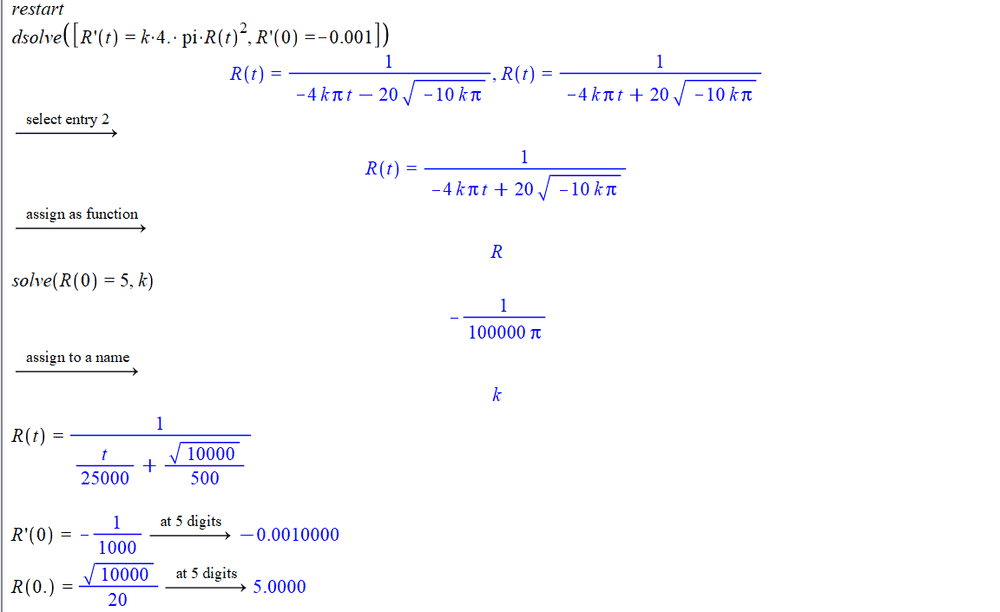# Solving a hard DE: R'(t)=k*4*pi*R(t)^2

• Mathman2013

#### Mathman2013

Homework Statement
solving the following ODE (Hard)
Relevant Equations
R'(t)=k*4*pi*R(t)^2
I have the following DE, R'(t) = k*4*pi*R(t)^2, where R(0) = 5, and R'(0) =-0.001. I have attempted to solve it Maple, and would like to know if I have done it correctly?Last edited by a moderator:
would like to know if I have done it correctly
The way to check that you (Maple?) have done it correctly is to differentiate twice and see if it matches !
You don't need PF to do that for you, do you?

 and what about entry 1 ?
[edit2] Strike the 'twice'.

##\ ##

Last edited:
The way to check that you (Maple?) have done it correctly is to differentiate twice and see if it matches !
You don't need PF to do that for you, do you?

 and what about entry 1 ?

##\ ##

I can't find k if I select entry 1.

How wold you go about if you had to solve this DE yourself ?

You mention 'hard' but it really isn't difficult ...

##\ ##

The way to check that you (Maple?) have done it correctly is to differentiate twice and see if it matches !
The DE is first order, so the OP needs only to differentiate the solution function once.

•BvU
This DE is solvable by hand. Notice that the original equation can be written as
dR/R^2 = Constant*dt

•Oops !sorry.